• 行业分类-电信-一种基于准平稳信号局部协方差匹配的KR子空间DOA估计方法.rar
• 通过最小化基于修正的协方差匹配准则来获得所提出的方法，该准则是通过根据正则化方法添加惩罚来获得的。此最小化问题显示为半定程序（SDP），并转换为约束二次规划问题，以降低计算复杂度，这可以通过增强拉格朗日...研究论文
•   通过对比残差协方差的在线估计值及其理论值来自适应调整过程噪声方差矩阵Qd\mathbf{Q}_{\mathrm{d}}Qd​以及测量噪声方差矩阵R\mathbf{R}R，直到残差协方差的估计值和理论值相等。   假定R\mathbf{R}R已知，...
1 原理
通过对比残差协方差的在线估计值及其理论值来自适应调整过程噪声方差矩阵

Q

d

\mathbf{Q}_{\mathrm{d}}

以及测量噪声方差矩阵

R

\mathbf{R}

，直到残差协方差的估计值和理论值相等。   假定

R

\mathbf{R}

已知，残差协方差的实际值为

A

^

=

1

N

∑

j

=

i

−

N

+

1

i

r

j

r

j

T

(1)

\widehat{\mathbf{A}}=\frac{1}{N} \sum_{j=i-N+1}^{i} \mathbf{r}_{j} \mathbf{r}_{j}^{\mathrm{T}}\tag{1}

与其相对应的理论值

[

H

(

t

i

)

P

(

t

i

−

)

H

T

(

t

i

)

+

R

(

t

i

)

]

\left[\mathbf{H}\left(t_{i}\right) \mathbf{P}\left(t_{i}^{-}\right) \mathbf{H}^{\mathrm{T}}\left(t_{i}\right)+\mathbf{R}\left(t_{i}\right)\right]

由卡尔曼滤波器提供。如果

A

^

\widehat{\mathbf{A}}

超过了滤波器推导的理论值(这个超过可以指特征值，对角元或者范数意义上)，那么过程噪声

Q

d

\mathbf{Q}_{\mathrm{d}}

就应该增加。具体方法如下。由

A

(

t

i

)

=

H

P

(

t

i

−

)

H

T

+

R

=

H

[

Φ

P

(

t

i

−

1

+

)

Φ

T

+

G

d

Q

d

G

d

T

]

H

T

+

R

\mathbf{A}\left(t_{i}\right)=\mathbf{H} \mathbf{P}\left(t_{i}^{-}\right) \mathbf{H}^{\mathrm{T}}+\mathbf{R}=\mathbf{H}\left[\mathbf{\Phi} \mathbf{P}\left(t_{i-1}^{+}\right) \mathbf{\Phi}^{\mathrm{T}}+\mathbf{G}_{\mathrm{d}} \mathbf{Q}_{\mathrm{d}} \mathbf{G}_{\mathrm{d}}^{\mathrm{T}}\right] \mathbf{H}^{\mathrm{T}}+\mathbf{R}

可得

H

G

d

Q

d

G

d

T

H

T

=

A

(

t

i

)

−

H

Φ

P

(

t

i

−

1

+

)

Φ

T

H

T

−

R

(2)

\mathbf{H G}_{\mathrm{d}} \mathbf{Q}_{\mathrm{d}} \mathbf{G}_{\mathrm{d}}^{\mathrm{T}} \mathbf{H}^{\mathrm{T}}=\mathbf{A}\left(t_{i}\right)-\mathbf{H} \mathbf{\Phi} \mathbf{P}\left(t_{i-1}^{+}\right) \mathbf{\Phi}^{\mathrm{T}} \mathbf{H}^{\mathrm{T}}-\mathbf{R}\tag{2}

具体求解

Q

d

\mathbf{Q}_{\mathrm{d}}

可能需要借助伪逆。   如果

Q

d

\mathbf{Q}_{\mathrm{d}}

已知，则

R

\mathbf{R}

可以用下式进行估计

R

^

=

1

N

∑

j

=

i

−

N

+

1

i

r

j

r

j

T

−

H

P

(

t

i

−

)

H

T

(3)

\hat{\mathbf{R}}=\frac{1}{N} \sum_{j=i-N+1}^{i} \mathbf{r}_{j} \mathbf{r}_{j}^{\mathrm{T}}-\mathbf{H} \mathbf{P}\left(t_{i}^{-}\right) \mathbf{H}^{\mathrm{T}}\tag{3}

2. 应用
其中一种方法是把待估计的过程噪声方差矩阵建模为

Q

d

=

Q

d

0

+

a

[

Δ

Q

d

]

(4)

\mathbf{Q}_{\mathrm{d}}=\mathbf{Q}_{\mathrm{d} 0}+a\left[\mathbf{\Delta Q}_{\mathrm{d}}\right]\tag{4}

其中，

Q

d

0

\mathbf{Q}_{\mathrm{d} 0}

是标称值，

Δ

Q

d

\Delta \mathbf{Q}_{\mathrm{d}}

是围绕标称值的小量，

a

a

是待调整参数。如果

a

a

有有限个备选选项，则该问提会被转化为一个多模型滤波问题。不同滤波器的

a

a

不同，结果选残差特性与理论值符合最好的

a

a

所对应的滤波器结果。
心得，评注
(1)式计算残差的实际协方差时，假定残差的期望是零。参考文献则去实际计算残差的期望，因此更符合真实实际情况。其具体公式为

W

^

k

=

1

k

∑

i

=

1

k

e

~

i

e

~

i

T

,

with

e

~

i

=

e

i

−

e

‾

i

(5)

其中

e

ˉ

i

=

1

i

∑

j

=

1

i

e

j

(6)

\bar{e}_{i}=\frac{1}{i} \sum_{j=1}^{i} e_{j}\tag{6}

为

i

i

时刻残差期望的估计值。公式(6)表明，对于

i

i

时刻残差期望的估计，使用==

i

i

时刻及以前的所有残差的平均值==。
参考文献
Stochastic models, estimation, and control VOLUME 2 10.10小节Motion and Parameter Estimation of Space Objects Using Laser-Vision Data
展开全文• 通过通用秩协方差矩阵匹配提高匹配场源本地化的统计稳健性研究论文
•研究论文
•研究论文
•研究论文
• 针对灰度及红外图像的匹配过程中经常出现的一些问题,如缺乏丰富的目标特征、易遭受复杂背景及噪声等外界因素干扰、目标出现放大缩小或偏转等,抽取目标图像的梯度幅值与方向,腐蚀与膨胀以及信息熵等特征,通过协方差...
• 协方差矩阵具有融合多维特征,获得全局最优解的优点,在目标描述方面展现出了优秀的性能,然而传统的协方差匹配难以跟踪被严重遮挡的目标,且全局搜索易遭受相似背景的干扰。为了提高协方差跟踪的性能,提出了基于遗忘...
• 自动构建常染色体遗传协方差矩阵和共享段列表，将前 n 个匹配项与 GEDmatch 试剂盒编号 在撰写本文时，此脚本可用于在 GEDmatch 网站上自动进行一对一和一对多的比较。 这些比较用于识别与感兴趣的人的前 n 个匹配...Python
• 最后,利用匹配滤波器的输出信杂比损失及相应的ANMF检测性能,对所提方法和已有方法进行效果评估,结果表明,本文方法具有收敛速度快、所需的辅助单元数少、信杂比损失小和对应的ANMF检测性能优等特点。
• 协方差 pythonThere is always a confusion when you are pursuing Statistical concepts. Let us see Covariance and Correlation in detail in this article. 当您追求统计概念时，总是会感到困惑。 让我们在本文...

协方差 python

There is always a confusion when you are pursuing Statistical concepts. Let us see Covariance and Correlation in detail in this article.
当您追求统计概念时，总是会感到困惑。 让我们在本文中详细了解协方差和相关性。
First useful information you can gather from data is how two variables are associated to each other. In layman terms, how one variable is related to other variable?
您可以从数据中收集的第一个有用信息是两个变量如何相互关联。 用外行术语来说，一个变量与另一个变量有什么关系？

Covariance and correlation are two significantly used terms in the field of statistics and probability theory. Both the terms measure the relationship and the dependency between two variables
协方差和相关性是统计学和概率论领域中两个重要使用的术语。 这两个术语都测量两个变量之间的关系和依赖性

Co-Variance
协方差
Covariance indicates the direction of the linear relationship between variables. Covariance values are not standard (…can be any number)
协方差指示变量之间线性关系的方向。 协方差值不是标准的(...可以是任何数字)
Covariance is given by formula -
协方差由公式给出-

Correlation
相关性
Correlation measures both the strength and direction of the linear relationship between two variables. Correlation values are standardized.
关联度测量两个变量之间线性关系的强度和方向。 相关值是标准化的。
Correlation is given by formula -
相关性由公式给出-
Co-relation coefficient formula

We can quantify the strength of the relationship with correlation. Weak correlation have small correlation value, where as strong correlation have larger value. You can obtain the correlation coefficient of two variables by dividing the covariance of these variables by the product of the standard deviations of the same values. When you divide the covariance values by the standard deviation, it essentially scales the value down to a limited range of -1 to +1. Correlation describes relationships and is not sensitive to the scale of data.
我们可以量化具有相关性的关系的强度。 弱相关具有较小的相关值，而强相关具有较大的值。 您可以通过将两个变量的协方差除以相同值的标准偏差的乘积来获得两个变量的相关系数。 当您用标准偏差除以协方差值时，它实际上将值缩小为-1到+1的有限范围。 关联描述关系，并且对数据规模不敏感。

Now let us see few differences between Covariance and Correlation -
现在让我们看一下协方差和相关之间的区别-
Difference between Covariance and Correlation

Now let us calculate and understand with an example, so it stays in our mind.
现在让我们通过一个示例进行计算和理解，因此它始终存在。
Let us take a simple example : X and Y are two variables
让我们举一个简单的例子：X和Y是两个变量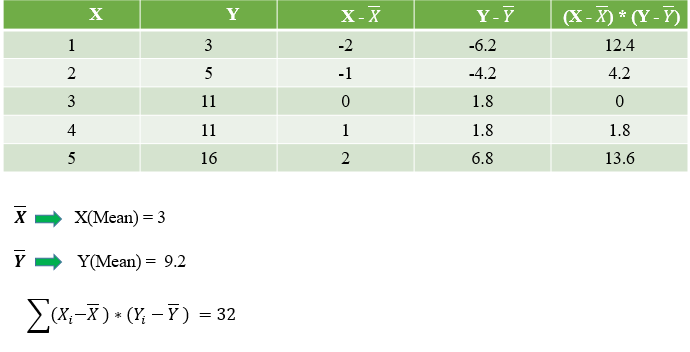Substituting in Co-Variance equation, we get,
代入协方差方程，我们得到，
Co-Variance of Sample is 8

Let us verify this in Python –
让我们用Python验证这一点–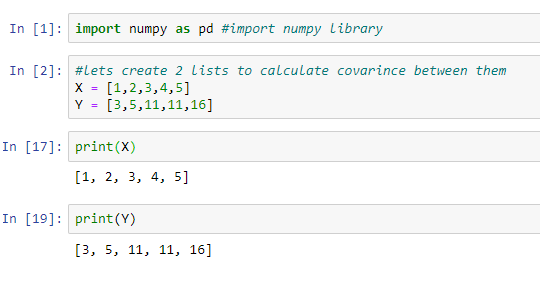Our Calculation and Python Calculation are matching

Now let us calculate Co-relation,
现在让我们计算相关
Substituting in above equation we get Sx and Sy as 1.58 and 5.21

Substituting in Co-relation equation, we get -
代入互相关方程，我们得到-
Co-relation Co-eff (r) = 0.9701

Let us verify this in Python –
让我们用Python验证这一点–
Manual Calculation and Python’s function results are matching

Conclusion –
结论–
We understood co-variance and correlation separately. We experimented using an example. We then compared with Python code.
我们分别了解协方差和相关性。 我们使用一个示例进行了实验。 然后，我们将其与Python代码进行了比较。
We got co-variance value as 8, which is a positive number (can be any positive infinity). And we achieved correlation coefficient as 0.97 which is clearly a strong positive correlation between X and Y.
我们得到的协方差值为8，这是一个正数(可以是任何正无穷大)。 并且我们获得了0.97的相关系数，这显然是X和Y之间的强正相关。

翻译自: https://medium.com/analytics-vidhya/covariance-and-correlation-math-and-python-code-7cbef556baed

协方差 python

展开全文python 算法
• 提出协方差矩阵结构的融合估计方法,通过调整参数涵盖现有的3种杂波协方差矩阵估计方法,并分析所提出方法对应的自适应归一化匹配滤波器的自适应特性.其次,确定了控制参数的经验公式,经验公式符合数值结果.最后,从估计...
• 该算法综合利用了区域协方差描述子维数较低, 量子遗传算法收敛速度快且全局寻优能力强和快速协方差交叉算法快速计算的特点, 能极大地提高了融合、匹配与更新过程的运算效率, 实现了快速有效的多特征融合跟踪。...
• 今天主要介绍一下MNE中如何用协方差矩阵来处理脑电数据的。MNE中的许多方法，包括源估计和一些分类算法，都需要根据记录进行协方差估计。在本教程中，我们将介绍传感器协方差计算的基础知识，...今天主要介绍一下MNE中如何用协方差矩阵来处理脑电数据的。
MNE中的许多方法，包括源估计和一些分类算法，都需要根据记录进行协方差估计。
在本教程中，我们将介绍传感器协方差计算的基础知识，并构建一个噪声协方差矩阵，该矩阵可用于计算最小范数逆解.
诸如MNE的源估计方法需要从记录中进行噪声估计。
在本教程中，我们介绍了噪声协方差的基础知识，并构造了一个噪声协方差矩阵，该矩阵可在计算逆解时使用。
下面我们将结合代码来进行分析。
# 导入工具库
import os.path as op

import mne
from mne.datasets import sample
import matplotlib.pyplot as plt

读取数据
# 构建数据地址
data_path = sample.data_path()
raw_empty_room_fname = op.join(
data_path, 'MEG', 'sample', 'ernoise_raw.fif')
raw_fname = op.join(data_path, 'MEG', 'sample', 'sample_audvis_raw.fif')
# 读取原始数据
#设置平均参考
raw.set_eeg_reference('average', projection=True)
#增加一个不良通道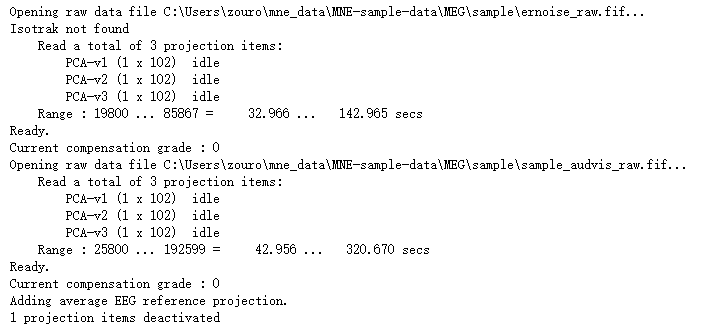噪声的定义取决于范式。在MEG中，通常使用空房间测量来估计传感器噪声。但是，如果要处理诱发的反应，可能还需要考虑将静息状态的大脑活动视为噪音。首先，我们使用空房间记录来计算噪声。请注意，您还可以仅将录制的一部分与tmin和tmax参数一起使用。如果您将静息状态用作噪声基线，这这将非常有用。在这里，我们使用整个空房间记录来计算噪声协方差。
请记住，在操作时要在处理方面将空房间数据集与实际的MEG数据进行匹配。确保过滤器都相同，并且如果使用ICA，则将其等效地应用于空房间和主题数据。在这种情况下，我们没有过滤数据，也没有使用ICA。但是，我们在MEG数据中确实存在错误的通道和投影，因此，我们要确保将它们存储在协方差对象中。
raw_empty_room.info['bads'] = [
bb for bb in raw.info['bads'] if 'EEG' not in bb]
[pp.copy() for pp in raw.info['projs'] if 'EEG' not in pp['desc']])
# 进行协方差均在计算
noise_cov = mne.compute_raw_covariance(
raw_empty_room, tmin=0, tmax=None)

3 projection items deactivated
Using up to 550 segments
Number of samples used : 66000
[done]
现在，已经在MNE-Python对象中有了协方差矩阵，可以使用mne.write_cov()将其保存到文件中。
还可以使用刺激前的基线来估计噪声协方差。
首先，我们必须构建epoch。
计算协方差时，应该在构建epochs时使用基线校正。否则协方差矩阵将不准确。
在MNE中，默认情况下会完成此操作，但为了确定，我们在此处手动定义它。
# 在原始数据中找到events
events = mne.find_events(raw)
# 构建epoch
epochs = mne.Epochs(raw, events, event_id=1, tmin=-0.2, tmax=0.5,
baseline=(-0.2, 0.0), decim=3,  # we'll decimate for speed
verbose='error')  # and ignore the warning about aliasing

320 events found
Event IDs: [ 1  2  3  4  5 32]
"""
对epochs数据进行协方差估计
"""
noise_cov_baseline = mne.compute_covariance(epochs, tmax=0)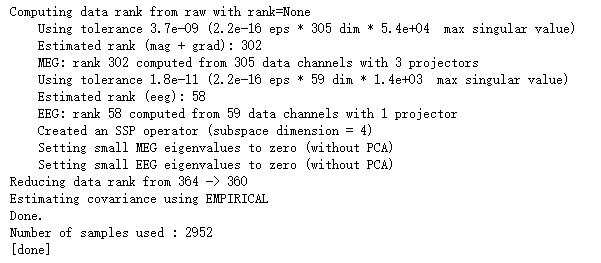绘制协方差矩阵
尝试将proj设置为False以查看效果。请注意，epochs中的投影机已经应用，因此proj参数无效。
noise_cov.plot(raw_empty_room.info, proj=True)

plt.show()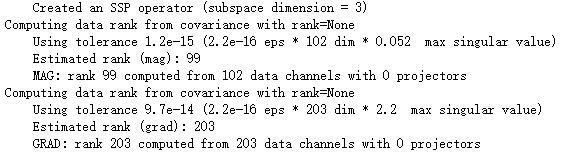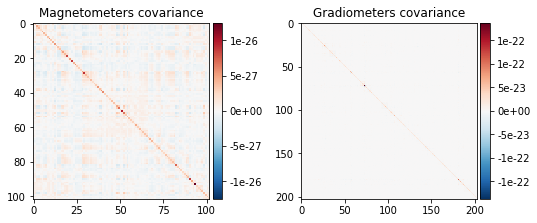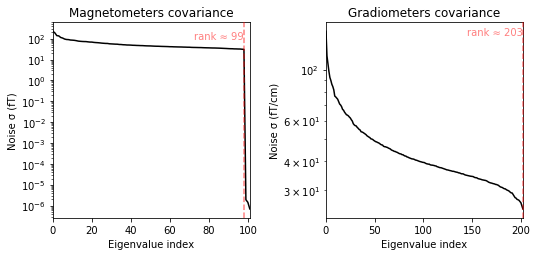noise_cov_baseline.plot(epochs.info, proj=True)
plt.show()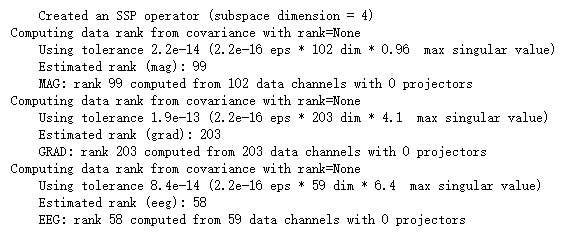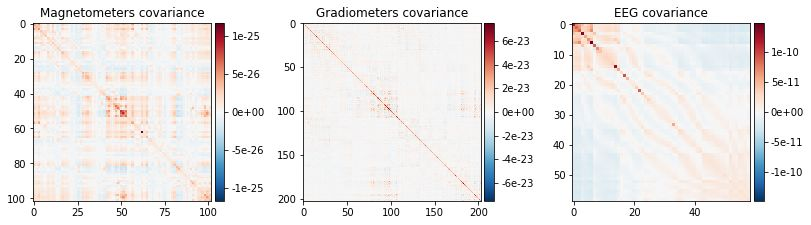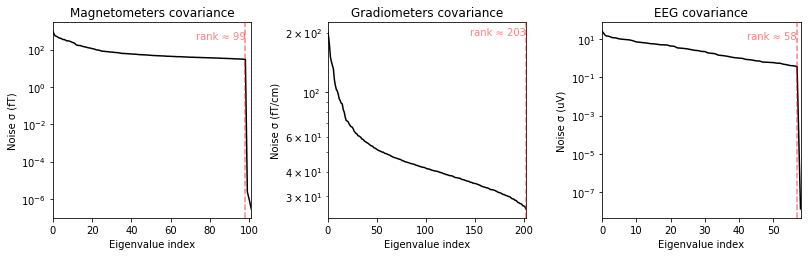应该如何规范协方差矩阵？
估计的协方差可能在数值上不稳定，并且倾向于在估计的源振幅和可用样本数之间引起相关性。
因此，MNE手册建议对噪声协方差矩阵进行正则化（请参阅对噪声协方差矩阵进行正则化），尤其是在只有少量样本可用的情况下。
然而，要说出样本的有效数量并不容易，因此要选择适当的正则化。
在MNE-Python中，使用中所述的高级正则化方法来完成正则化。为此，可以使用'auto'选项。使用此选项，交叉验证将用于学习最佳正则化：
noise_cov_reg = mne.compute_covariance(epochs, tmax=0., method='auto',
rank=None)

此过程使用看不见的数据的负对数似然来量化噪声协方差，从而量化噪声协方差。最终结果也可以用肉眼检查。在假设基线不包含系统信号(对感兴趣的事件进行时间锁定)的情况下，白化基线信号应遵循多元高斯分布，即，在给定的条件下，白化基线信号应在-1.96和1.96之间 时间样本。基于相同的推理，全局场功率(GFP)的期望值为1(计算GFP时应考虑真实的自由度，如ddof=3，其中有2个活动的SSP向量):
evoked = epochs.average()
evoked.plot_white(noise_cov_reg, time_unit='s')
plt.show()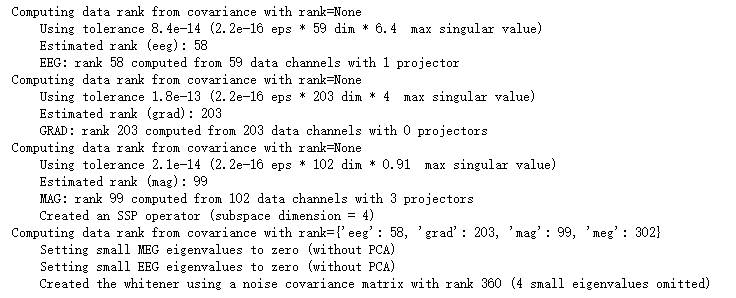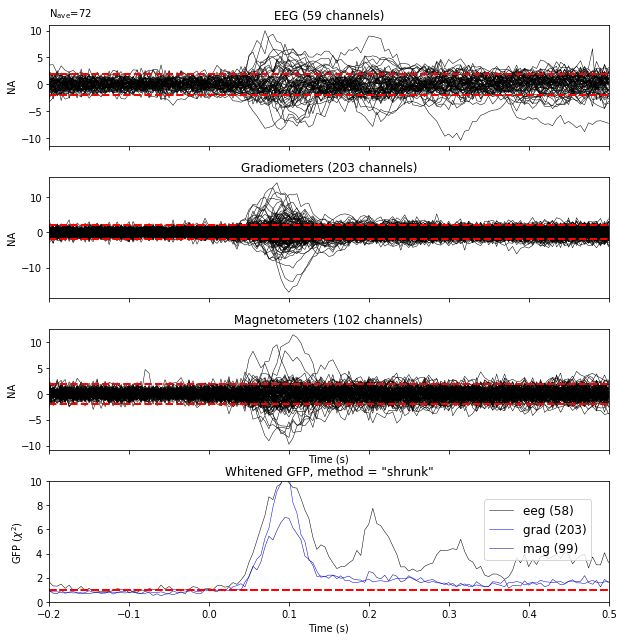该图同时显示了每个通道的白化诱发信号和白化的GFP。GFP面板中的数字代表数据的估计等级，相当于计算白色GFP时跨传感器的平方和除以的有效自由度。变白的GFP还有助于检测虚假的晚期诱发成分，这可能是由于过度或欠正则化所致。
请注意，如果使用信号空间分离(SSS) 2处理数据，则会同时显示梯度仪和磁力仪，因为两者都是由相同的SSS基向量以相同的数值秩重建的。这也意味着这两种传感器类型不再是统计上独立的。这些评估方法可用于评估模型违规。
对于专家用例或调试，还可以将替代估计量进行比较，并演示白化对源估计的影响：
noise_covs = mne.compute_covariance(
epochs, tmax=0., method=('empirical', 'shrunk'), return_estimators=True,
rank=None)
evoked.plot_white(noise_covs, time_unit='s')
plt.show()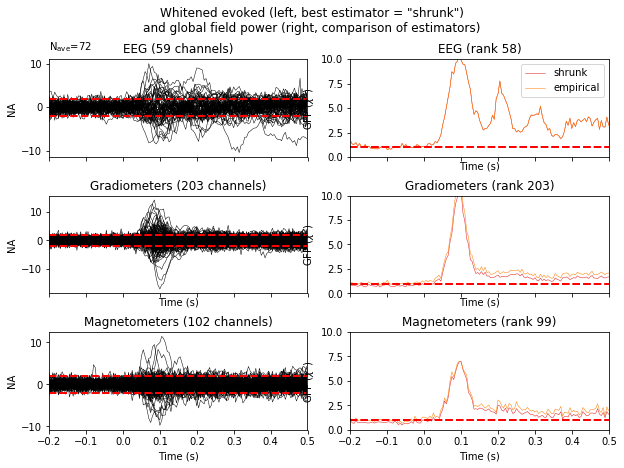这将绘制出为最佳估计量而诱发的白化并在相关面板中将所有估计器的GFP显示为单独的行。
最后，让我们看一下空房间和与事件相关的协方差之间的区别，使用"method"选项，使它们的类型显示在图例中。
evoked_meg = evoked.copy().pick('meg')
noise_cov['method'] = 'empty_room'
noise_cov_baseline['method'] = 'baseline'
evoked_meg.plot_white([noise_cov_baseline, noise_cov], time_unit='s')
plt.show()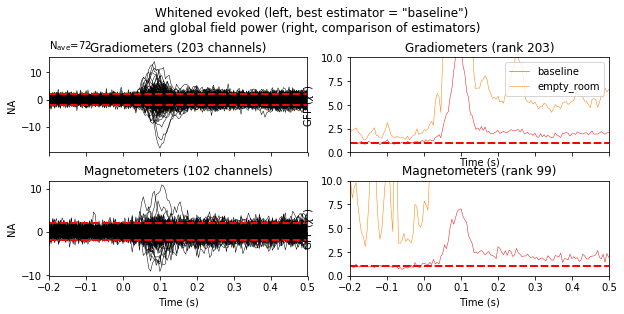基于对数可能性的负值，基线协方差似乎更合适。
参考
1.Engemann D. and Gramfort A. (2015) Automated model selection in covariance estimation and spatial whitening of MEG and EEG signals, vol. 108, 328-342, NeuroImage.
2.Taulu, S., Simola, J., Kajola, M., 2005. Applications of the signal space separation method. IEEE Trans. Signal Proc. 53, 3359-3372.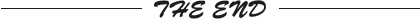不用于商业行为，转载请联系后台
若有侵权，请后台留言，管理员即时删侵！
更多阅读
EEG公开数据集汇总
CMU贺斌教授团队提出FAST-IRES技术
Python可视化Evoked数据
探索双耳助听器中的人体通信
手部的运动区域也与整个身体相连
他们想把广告植入你的梦境
EEG伪影详解和过滤工具的汇总(二)
投稿通道
如何让你的工作让更多人知晓和受益？
脑机接口社区就是这样一个连接学界、
企业界和爱好者的平台渠道。
社区鼓励高校实验室、企业或个人在我们平台上分享优质内容。
稿件要求
稿件系个人原创作品，若已在其他平台发表，请明确标注。
稿件一经录取，便提供稿费！
投稿通道
微信扫码，备注：投稿+姓名+单位微信交流群，请扫码上方微信
(备注：姓名+单位+专业/领域行业)
QQ交流群：913607986你的每一次在看，我都很在意！

展开全文人工智能 数据分析 python 数据挖掘 大数据
• 利用模糊理论和协方差匹配技术对扩展卡尔曼滤波算法中的观测噪声协方差R进行自适应调整，实现定位算法性能的在线改进；同时采用传感器故障诊断与修复算法来监测传感器的工作状态，提高定位算法的鲁棒性。将该方法...移动机器人定位 扩展卡尔曼滤波 模糊理论
• 样本均值和协方差与各自的总体矩匹配的多元正态模拟，请参阅A. Meucci (2009)，“具有精确均值和协方差的模拟”， 最新版本的文章和代码可在http://symmys.com/node/162 获得matlab
• 在评估结构方程模型的拟合，很常见的应用是研究...如果我们的模型的协方差矩阵实际上匹配抽样变异中的样本协方差矩阵，该χ2无论样本量多大，该检验在统计学上均无统计学意义。 因为到大样本量，从业人员往往依赖...
原文链接：http://tecdat.cn/?p=10426
原文出处：拓端数据部落公众号

在评估结构方程模型的拟合，很常见的应用是研究χ2进行测试，因为在给定足够大的样本量的情况下，它几乎总会检测出模型与数据之间的统计上的显着差异。因为，我们的模型几乎总是数据的近似值。如果我们的模型的协方差矩阵实际上匹配抽样变异中的样本协方差矩阵，该χ2 无论样本量多大，该检验在统计学上均无统计学意义。
因为到大样本量，从业人员往往依赖于其他拟合指数，如RMSEA，CFI和TLI-所有这些都是基于χ 2。在lavaan中，您会自动使用置信区间和p值对RMSEA进行紧密拟合测试。这个测试实际上使用χ2分布。

RMSEA的公式为：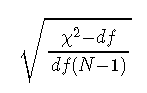其中，χ2是χ2模型的检验统计量，dF是模型自由度，N是样本量。
如果你的模型拟合数据完美，分子为零；这是标准的假设χ 2χ2-test测试。如果我们在RMSEA进行测试中，使用χ 2参数对应于RMSEA为0.05的分布。Lavaan将测试结果报告为拟合统计之一。
那么这对我们有什么帮助呢？非中心参数（λ ）在lavaan的RMSEA测试实际上是χ 2 - d ˚Fχ2-dF对应于RMSEA为0.05的值。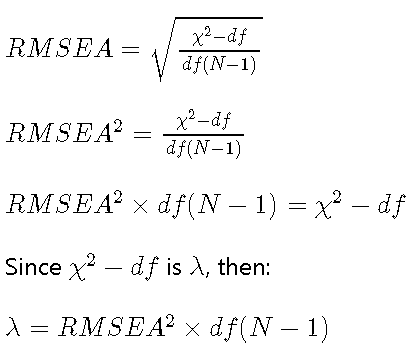因此，对于测试，λ 是：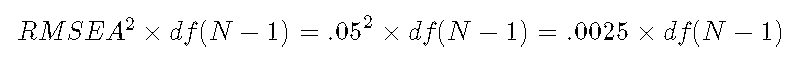对于中等拟合的测试，λ 是：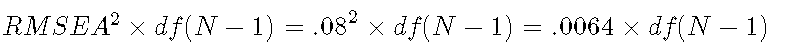请注意，lavaan的处理方式可能有所不同。
因此，给定模型的自由度和样本量，我们可以计算出非中心性参数（λ ）。给定λ中，χ2 值和模型的自由度，我们可以计算p值进行测试。
R的语法是：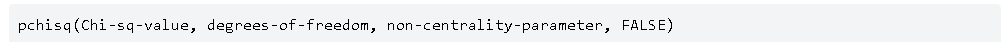示范 

# 具有HolzingerSwineford1939数据集的双因子模型的模型语法
writeLines(syntax <- paste(
paste("g =~", paste0("x", 1:9, collapse = " + ")),
# paste("visual =~", paste0("x", 1:3, collapse = " + ")),
paste("textual =~", paste0("x", 4:6, collapse = " + ")),
paste("speed =~", paste0("x", 7:9, collapse = " + ")),
sep = "\n"
))

g =~ x1 + x2 + x3 + x4 + x5 + x6 + x7 + x8 + x9
textual =~ x4 + x5 + x6
speed =~ x7 + x8 + x9
运行模型并报告拟合度。仅报告统计信息：


lavaan (0.5-23.1097) converged normally after  25 iterations

Number of observations                           301

Estimator                                         ML
Minimum Function Test Statistic               42.291
Degrees of freedom                                21
P-value (Chi-square)                           0.004

Root Mean Square Error of Approximation:

RMSEA                                          0.058
90 Percent Confidence Interval          0.032  0.083
P-value RMSEA <= 0.05                          0.276

卡方统计意义显着，该完美拟合检验表明，由于样本的变异性。
默认的卡方检验：
pchisq

 0.003867178

使用上面的公式计算紧密度测试的非中心参数：.0025乘以模型自由度乘以样本大小-1
 ncp.close

 15.75

计算紧密拟合的卡方检验：
pchisq

 0.2740353

紧密契合度测试的p值为.27，接近lavaan报告的值。
如果我们降低标准以进行中等拟合的卡方检验：.0064乘以模型自由度乘以样本大小-1
 ncp.med

 40.32

pchisq

 0.9199686

我们在模型中观察模型隐含的协方差矩阵的可能性为92％。非常好。

最后，SEM从业者通常报告χ 2-test，但通常希望该测试能够检测到模型规范错误，因此在实践中经常将其忽略。

PS：潜在变量建模的另一种方法是PLS路径建模。这是一种基于OLS回归的SEM方法。

MacCallum, R. C., Browne, M. W., & Sugawara, H. M. (1996). Power analysis and determination of sample size for covariance structure modeling. Psychological Methods, 1(2), 130–149. https://doi.org/10.1037/1082-989X.1.2.130 ↩最受欢迎的见解
1.Matlab马尔可夫链蒙特卡罗法（MCMC）估计随机波动率（SV，Stochastic Volatility） 模型
2.基于R语言的疾病制图中自适应核密度估计的阈值选择方法
3.WinBUGS对多元随机波动率模型：贝叶斯估计与模型比较
4.R语言回归中的hosmer-lemeshow拟合优度检验
5.matlab实现MCMC的马尔可夫切换ARMA – GARCH模型估计
6.R语言区间数据回归分析
7.R语言WALD检验 VS 似然比检验
8.python用线性回归预测股票价格
9.R语言如何在生存分析与Cox回归中计算IDI，NRI指标
展开全文R语言 结构方程 卡方检验
• 1、前两种都是属于模板匹配的方法，这些概念是在《数字图像处理高级应用》里的，其是移动匹配与向量匹配很像，只是移动匹配对灰度变换的鲁棒性不好。 这里说的移动匹配：就是把模板图像在原图像上进行移动，让后计算...
•自动驾驶 人工智能
• 由于匹配结果有时候只约束单个方向，比如法向，因此需要考虑只约束部分方向的误差方程写法，虽然可以使用自定义边，但经过测试，自定义欧式距离边加上位姿的求解通常不能收敛，因此这里均采用位姿的误差边形式，借用...
•算法
• 希望通过此题，让大家熟悉一下一些概念：均值/期望，方差，协方差，相关系数。 基本概念科普 期望 ， 方差 等被称作统计变量的数字特征。我们知道，概率密度函数可以完全描述一个统计变量的特性。正如一个用一...
• 自制影像匹配小软件。对左图和右图进行影像匹配。进行Moravec算子提取特征，而后以特征点为基础建立搜索区域，对待匹配点进行检测，利用协方差函数进行相似性测度的评定。 开发环境:Win7 64位+ vs2015/MFC
• 自制影像匹配小软件。对左图和右图进行影像匹配。进行Moravec算子提取特征，而后以特征点为基础建立搜索区域，对待匹配点进行检测，利用协方差函数进行相似性测度的评定。 开发环境:Win7 64位+ vs2015/MFC
• 在这个背景下,首先,根据协方差匹配原理建立一种带有过程噪声递推估计的自适应KF算法;然后,为了突破KF只能处理线性系统估计问题的局限,将过程噪声递推估计引入集合卡尔曼滤波(EnKF)中,提出一种自适应EnKF算法;最后,...
• 协方差与相关系数协方差与相关系数协方差相关系数1.协方差如果两个变量的变化趋势一致，也就是说如果其中一个大于自身的期望值时另外一个也大于自身的期望值，那么两个变量之间的协方差就是正值；如果两个变量的变化......